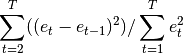# statsmodels.stats.stattools.durbin_watson¶

statsmodels.stats.stattools.durbin_watson(resids, axis=0)[source]

Calculates the Durbin-Watson statistic

Parameters: resids : array-like dw : float, array-like The Durbin-Watson statistic.

Notes

The null hypothesis of the test is that there is no serial correlation. The Durbin-Watson test statistics is defined as:The test statistic is approximately equal to 2*(1-r) where r is the sample autocorrelation of the residuals. Thus, for r == 0, indicating no serial correlation, the test statistic equals 2. This statistic will always be between 0 and 4. The closer to 0 the statistic, the more evidence for positive serial correlation. The closer to 4, the more evidence for negative serial correlation.